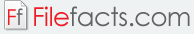### KAlgebra Mobile (Pocket Graph Calculator)

#### KDE (Open Source)

KAlgebra Mobile (Pocket Graph Calculator) is a mathematical graph calculator developed by KDE as part of its KDE Education Package. The program was first released in 2005. It is based on the content markup language, MathML. It is very user friendly, enabling even non-mathematically-adept users to understand how it functions.  The program features numerical, symbolic, logical, and analytic functions. Through the program, users can plot the results of a mathematical function into a 3D or 2D space.  The program is licensed under a GNU GPL or GNU General Public License.

KAlgebra Mobile (Pocket Graph Calculator) features a simple grey tabbed interface. The four main tabs are Console, 3D Graph, 2D Graph, and Dictionary. The program utilizes algebraic syntax to calculate the mathematical expressions entered into the program. This mathematical function can then be viewed using the 2D Graph or 3D Graph, which features a colorful and interactive interface. Calculations are performed using scripts and macros that are provided by the users. The 2D and 3D Graphing tool allows users to plot and evaluate functions. However, these graphs are largely dependent on the x and y functions. Both graphing tools allow users to define the viewpoint. As an added function, users can hover their mouse over any part of the line to find out the exact x and y values. The Dictionary tool, on the other hand, provides a comprehensive list of functions built into the program. This may include examples, formulas, parameters, and sample plots. The program features more than 100 functions and operations integrated into the program.